• +91 9971497814
• info@interviewmaterial.com

# Introduction to Trigonometry Ex-8.1 Interview Questions Answers

### Related Subjects

Question 1 :

In ∆ ABC,right-angled at B, AB = 24 cm, BC = 7 cm. Determine:

(i) sin A, cos A

(ii) sin C, cos C

Solution:

In a given triangle ABC, right angled at B = B = 90°

Given: AB = 24 cm and BC = 7 cm

According to the Pythagoras Theorem,

In a right- angled triangle, the squares ofthe hypotenuse side is equal to the sum of the squares of the other two sides.

By applying Pythagoras theorem, we get

AC2=AB2+BC2

AC2 = (24)2+72

AC2 = (576+49)

AC2 = 625cm2

AC = √625 = 25

Therefore, AC = 25 cm

(i) To find Sin (A), Cos (A)

We know that sine (or) Sin function is theequal to the ratio of length of the opposite side to the hypotenuse side. So itbecomes

Sin (A) = Opposite side /Hypotenuse = BC/AC =7/25

Cosine or Cos function is equal to the ratio ofthe length of the adjacent side to the hypotenuse side and it becomes,

Cos (A) = Adjacent side/Hypotenuse = AB/AC =24/25

(ii) To find Sin (C), Cos (C)

Sin (C) = AB/AC = 24/25

Cos (C) = BC/AC = 7/25

Question 2 :

In Fig. 8.13, findtan P – cot R

Answer 2 :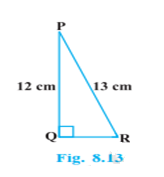Solution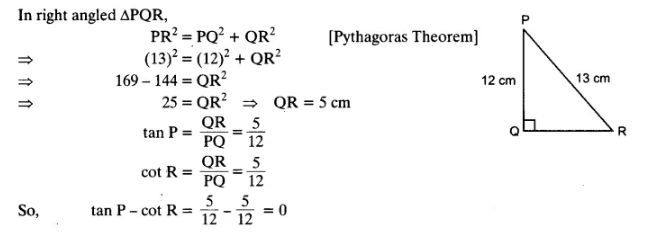Question 3 : If sin A = 3/4, Calculate cos A and tan A.

Answer 3 :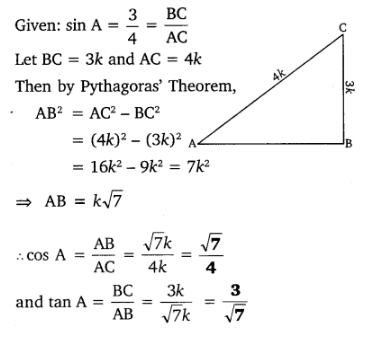Question 4 : Given 15 cot A = 8, find sin A and sec A.

Answer 4 :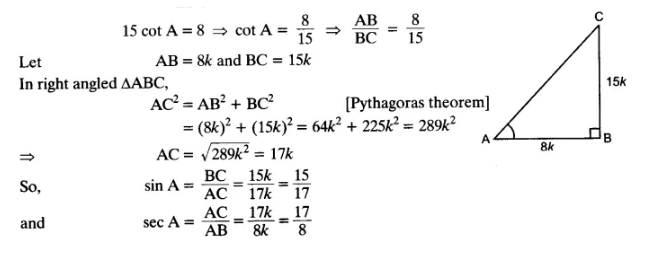Question 5 : Given sec θ = 13/12 Calculate all othertrigonometric ratios

Answer 5 :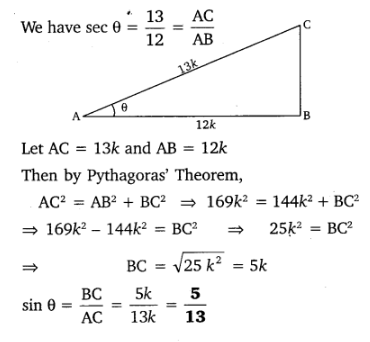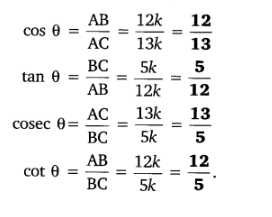Question 6 :

If A and B are acute angles such that cos A =cos B, then show that A = B.

Answer 6 :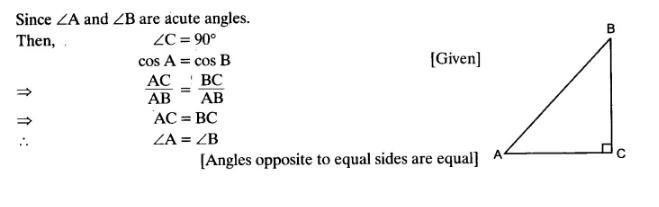Question 7 :

If cot θ = 7/8,evaluate :

(i) (1 + sin θ)(1 – sin θ)/(1+cos θ)(1-cos θ)

(ii) cot_2 θ

Solution:

Let us assume a ABC in which B = 90° and C = θ

Given:

cot θ = BC/AB = 7/8

Let BC = 7k and AB = 8k, where k is a positivereal number

According to Pythagoras theorem in ABC we get.

AC= AB2+BC2

AC= (8k)2+(7k)2

AC= 64k2+49k2

AC= 113k2

AC = √113 k

According to the sine and cos function ratios,it is written as

sin θ = AB/AC = Opposite Side/Hypotenuse =8k/√113 k = 8/√113 and

cos θ = Adjacent Side/Hypotenuse = BC/AC =7k/√113 k = 7/√113

Now apply the values of sin function and cosfunction: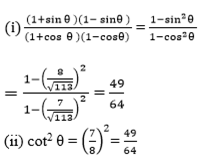Question 8 :

If 3 cot A = 4,check whether (1-tanA)/(1+tan2 A) = cos2 A– sin A or not.

Let ABC inwhich B=90°

We know that, cot function is the reciprocalof tan function and it is written as

cot(A) = AB/BC = 4/3

Let AB = 4k an BC =3k, where k is a positivereal number.

According to the Pythagorean theorem,

AC2=AB2+BC2

AC2=(4k)2+(3k)2

AC2=16k2+9k2

AC2=25k2

AC=5k

Now, apply the values corresponding to theratios

tan(A) = BC/AB = 3/4

sin (A) = BC/AC = 3/5

cos (A) = AB/AC = 4/5

Now compare the left hand side(LHS) with righthand side(RHS)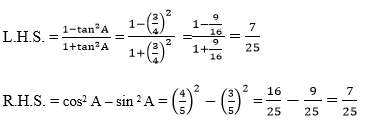Since, both the LHS and RHS = 7/25

R.H.S. =L.H.S.

Hence, (1-tanA)/(1+tan2 A) = cos2 A– sin A  is proved

Question 9 :

In triangle ABC,right-angled at B, if tan A = 1/√3 find the value of:

(i) sin A cos C + cos A sin C

(ii) cos A cos C – sin A sin C

Solution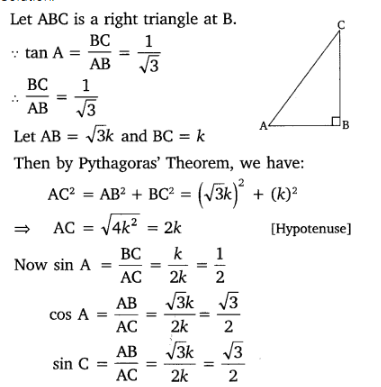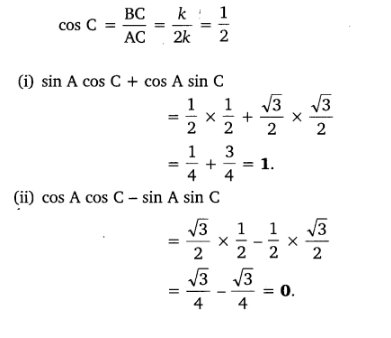Question 10 :

In ∆ PQR,right-angled at Q, PR + QR = 25 cm and PQ = 5 cm. Determine the values of sinP, cos P and tan P

Answer 10 :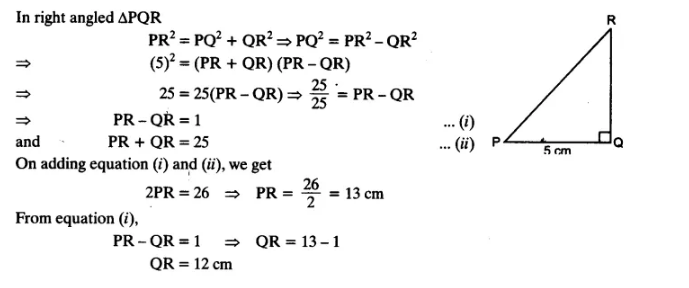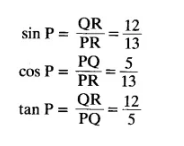Todays Deals